Math resources Geometry Angles

Supplementary angles

# Supplementary angles

Here you will learn about supplementary angles, including how to find missing angles by applying knowledge of supplementary angles.

Students will first learn about supplementary angles as a part of measurement and data in 4th grade. They will expand that knowledge as they progress through middle school.

## What are supplementary angles?

Supplementary angles are two angles that add up to 180 degrees. They can be adjacent or not adjacent. Adjacent angles are angles that share a common arm and a vertex.

Adjacent supplementary angles make a straight line.

You can use what you know about supplementary angles to decompose angles and find the measurement of an unknown angle.

### What are supplementary angles?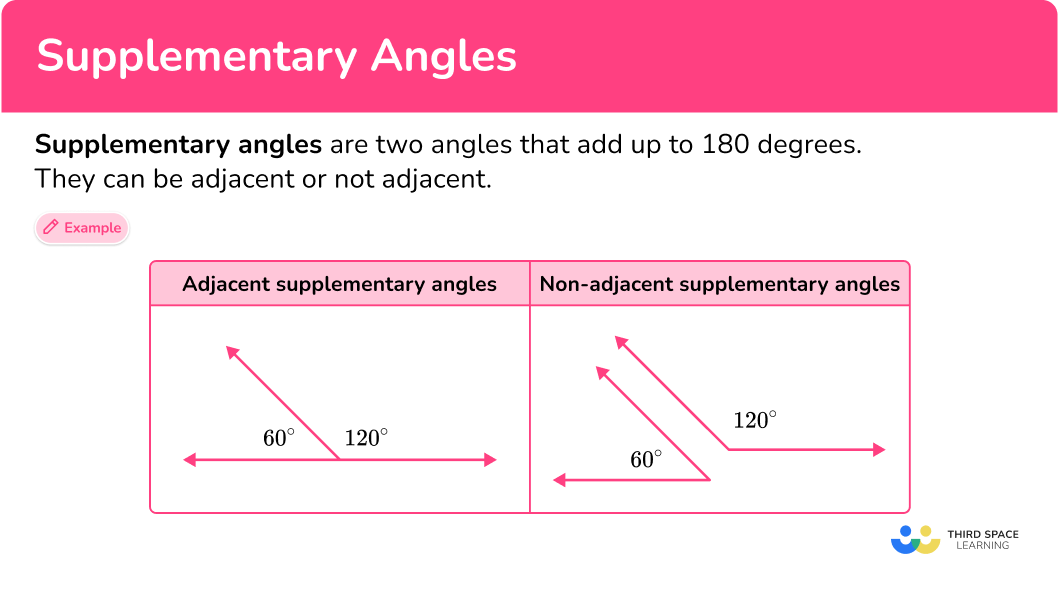## Common Core State Standards

How does this relate to 4th grade math?

• Grade 4: Measurement and Data (4.MD.C.7)
Recognize angle measure as additive. When an angle is decomposed into non-overlapping parts, the angle measure of the whole is the sum of the angle measures of the parts. Solve addition and subtraction problems to find unknown angles on a diagram in real world and mathematical problems, for example, by using an equation with a symbol for the unknown angle measure.

## How to find the measure of supplementary angles

In order to find the measure of supplementary angles you need to:

1. Determine which angles are supplementary.
2. Identify given angle measurements and the unknown angle or angles.
3. Find the missing angle.

## Supplementary angles examples

### Example 1: finding missing angle measures (adjacent angles)

The two angles shown are supplementary. Find the measure of angle x.

1. Determine which angles are supplementary.

The question states that angles x and y are supplementary and equal 180^{\circ}.

x+y=180

2Identify given angle measurements and the unknown angle or angles.

Angle y is 17^{\circ}.

The unknown angle is angle x, and when it’s added to 17^{\circ} will equal 180^{\circ}.

3Find the missing angle.

To find the missing angle, you will subtract 17 from 180.

x=180-17

x = 163

4Clearly state the answer using angle terminology.

The measurement of angle x is 163^{\circ}.

### Example 2: finding missing angle measures (adjacent angles)

\angle ABC and \angle CBD are supplementary angles. \angle ABC has a measure of 109^{\circ}. What is the measure of \angle CBD?

Determine which angles are supplementary.

Identify given angle measurements and the unknown angle or angles.

Find the missing angle.

### Example 3: finding missing angle (non-adjacent angles)

The pair of angles are supplementary. Find the measure of angle F.

Determine which angles are supplementary.

Identify given angle measurements and the unknown angle or angles.

Find the missing angle.

### Example 4: finding missing angle (not adjacent)

\angle L and \angle M are supplementary angles. \angle M measures at 98^{\circ}. What is the measure of \angle L?

Determine which angles are supplementary.

Identify given angle measurements and the unknown angle or angles.

Find the missing angle.

### Example 5: finding missing angle (no diagram)

Two angles ‘x and y' are supplementary and one of them is 147^{\circ}. What is the size of the other angle?

Determine which angles are supplementary.

Identify given angle measurements and the unknown angle or angles.

Find the missing angle.

### Example 6: finding missing angle

Two angles ‘x and y' are supplementary and one of them is a right angle. What is the size of the other angle?

Determine which angles are supplementary.

Identify given angle measurements and the unknown angle or angles.

Find the missing angle.

### Teaching tips for supplementary angles

• Rather than having students practice finding and decomposing supplementary angles on multiple skill worksheets, provide them with a variety of practice problems, activities, and/or projects that have a real-world context. This will deepen their understanding of this skill.

### Easy mistakes to make

• Mixing up supplementary angles and complementary angles
Students may mix up supplementary and complementary angles, thinking that complementary angles sum to 180^{\circ} and that supplementary angles sum to 90^{\circ}. However, complementary angles have a sum of 90^{\circ} and supplementary angles have a sum of 180^{\circ}.

• Assuming supplementary angles must have a common vertex
Supplementary angles can be either adjacent or not adjacent. Not adjacent supplementary angles do not share a common vertex, but their angles will still add up to 180^{\circ}.

### Practice supplementary angles questions

1. The two angles shown are supplementary. Find the measure of \angle pmn.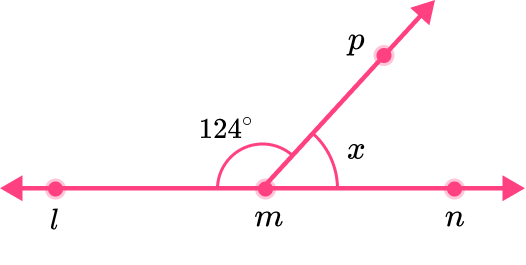x=112^{\circ}x=56^{\circ}x=106^{\circ}x=65^{\circ}The two angles are supplementary, so they must have a sum of 180.

You will subtract 124 from 180 to find the measure of the missing angle.

x=180-124

x=56

The missing angle measures at 56^{\circ}.

2. The two angles shown are supplementary. Which of the following could be the measure of \angle srt?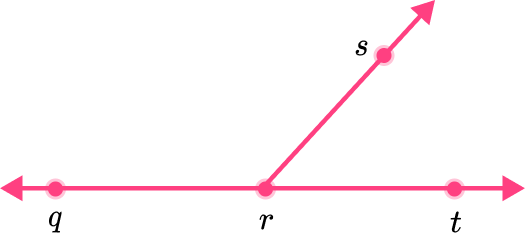\angle srt=45^{\circ}\angle srt=114^{\circ}\angle srt=90^{\circ}\angle srt=10^{\circ}\angle srt is an acute angle, which means that it’s measurement is less than 90^{\circ}.

An angle with the measure of 114^{\circ} would be an obtuse angle. \angle srt can not equal 114^{\circ}.

An angle with the measure of 90^{\circ} would be a right angle. \angle srt is not a right angle.

An angle with the measure of 10^{\circ} would be an acute angle, however, it would be a very skinny acute angle. \angle srt is too large to equal 10^{\circ}.

\angle srt is an acute angle, with a measurement of 45^{\circ}.

2. \angle a and \angle b are supplementary angles. \angle a measures at 14^{\circ}. What is the measure of \angle b?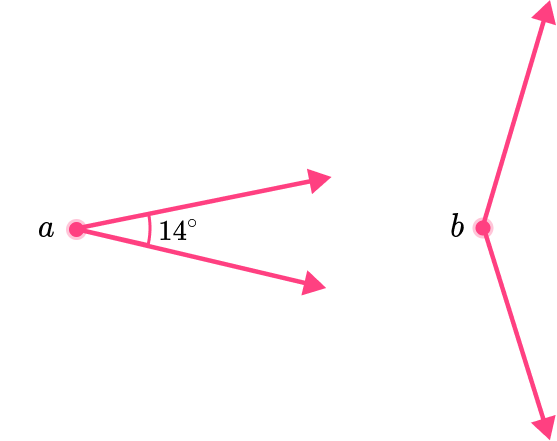b=76^{\circ}b=166^{\circ}b=83^{\circ}b=114^{\circ}The two angles are supplementary, so they must have a sum of 180.

You will subtract 14 from 180 to find the measure of the missing angle.

b=180-14

b=166

The missing angle measures at 166^{\circ}.

4. \angle f and \angle g are supplementary angles. \angle f measures at 113^{\circ}. What is the measure of \angle g?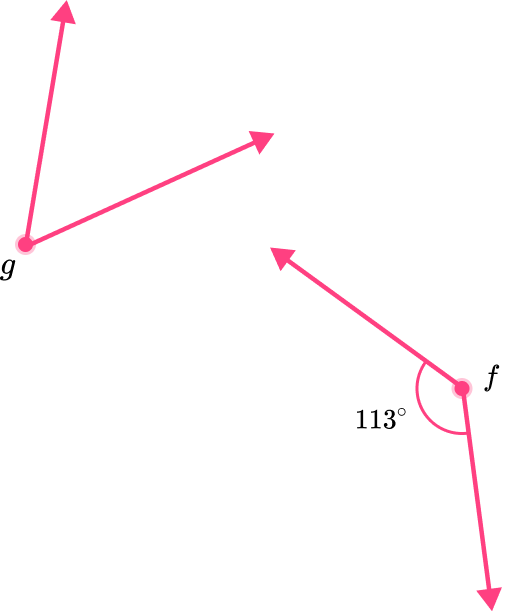113^{\circ}23^{\circ}77^{\circ}67^{\circ}The two angles are supplementary, so they must have a sum of 180.

You will subtract 113 from 180 to find the measure of the missing angle.

g=180-113

g=67

The missing angle measures at 67^{\circ}.

5. Two angles 'x and y' are supplementary and one of them is 47^{\circ}. What is the size of the other angle?

43^{\circ}133^{\circ}47^{\circ}313^{\circ}The two angles are supplementary, so they must have a sum of 180.

You will subtract 47 from 180 to find the measure of the missing angle.

x=180-47

x=133

The missing angle measures at 133^{\circ}.

6. Two angles 'x and y' are supplementary and one of them is 123^{\circ}. What is the size of the other angle?

53^{\circ}123^{\circ}57^{\circ}237^{\circ}The two angles are supplementary, so they must have a sum of 180.

To find the measure of the missing angle, you can subtract 123 from 180.

x=180-123

x=57

The missing angle measures at 57^{\circ}.

## Supplementary angles FAQs

What’s the difference between complementary and supplementary angles?

Supplementary angles add up to 180 degrees, or a straight angle.
Complementary angles add up to 90 degrees, or a right angle.

Can three angles be supplementary?

No, three angles will never be supplementary, even if the sum of their measurement is 180^{\circ}. Supplementary angles will only occur in pairs.

Is there a formula for supplementary angles?

\angle a + \angle b = 180^{\circ}; two angles are supplementary if angle \; a and angle \; b equal 180^{\circ}.

## Still stuck?

At Third Space Learning, we specialize in helping teachers and school leaders to provide personalized math support for more of their students through high-quality, online one-on-one math tutoring delivered by subject experts.

Each week, our tutors support thousands of students who are at risk of not meeting their grade-level expectations, and help accelerate their progress and boost their confidence.